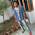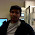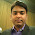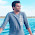# Probability - Shortcuts and Questions with Answers

Probability is one of the important chapter for IBPS and other competitive exams like CAT. Questions in this chapter are relatively easier than other chapters. Most of the time set of 4-5 questions is asked in competitive exams which can be solved in minutes. I have tried my best to provide shortcut tricks for my readers. In case you find any problem then please comment below. Before reading this article read Permutation and Combination concept.

### My previous posts on Quantitative aptitude :-

Time and Work
Data Sufficiency

Probability = Occurrence of event/Total possible events

## Simple probability problem

Simplest questions asked in bank exams are asked about deck of cards, dice and coins. There is no co-occurrence of events.

Lets take an example :-

Question -One card is drawn from a deck of 52 cards. Find the probability that it is a black queen or king.

Simple solution to this problem is 2/52 = 1/26

## Venn Diagram

Question - One card is drawn from a deck of 52 cards. Each card is equally likely to be drawn. Find the probability that card drawn is either black or queen.

In this question we are going to use a simple formula

P(AB) = A ∪ B ∩ AB

Don't be confused with the formula. Give a look to this diagram

In a deck of cards there are 26 black cards and 4 queens. But 2 black cards are queen. These cards are part of grey and brown circles in above diagram. As pink region is added twice, we need to deduct it once.

So the solution is  26 + 4 - 2 = 28

Probability (AB) = 28/52 = 7/13

## Independent Events

In case you need to find probability of happening of two independent events we use multiplication theorem.

P(AB) = P(A) * P(B)

Lets understand this theorem with help of an example

Question - An unbiased coin is tossed twice. Find the probability of getting 1,2,3 or in the first toss and 4,5 or 6 in second attempt ?

Solution - Probability of getting 1,2,3 or 4 in first toss = 4/6 = 2/3
Probability of getting 4,5 or 6 in second toss = 3/6 = 1/2

Now both events are independent and we will use multiplication theorem

1/2 * 2/3 = 1/3

## Important Probability questions set - Venn diagram

This question is frequently asked in the exams. Even in IBPS 2012 and SBI 2013, this question appeared with slight difference in amounts. Still most of the candidates are unable to solve this.

Question - There are 200 students in commerce batch of Khalsa college. Out of them 100 play cricket, 50 play hockey and 60 play basketball. 3o students play both cricket and hockey, 45 students play both cricket and basketball and 35 students play both hockey and basketball.

1) What is the maximum number of students who play all the games ?
2) What is minimum number of students who play all three games ?
3) What is the maximum number of students who play at least one game ?
4) What is the minimum number of students playing at least one game ?

Solution - To make the question simple, draw a Venn diagram and let x = number of students who play all games. Complete the diagram.

Solution 1
What is the maximum value of x ?
Consider equation 30 - x, it states that maximum value of x = 30
In case x>30, for example 32 then 30 - x will be negative and number of students can't be negative. So answer is 30.

Solution 2
What is the minimum value of x ?
consider x-20 is possible as negative value of students is not possible. It is possible to deduct 20 from x.
So minimum value of x = 20

Solution 3 and 4
For 3rd and 4th questions we will add everything :-

25 + x + x - 15 + x - 20 + 45 - x  + 35 - x + 30 - x + x =
= 100 + x
Here we add maximum and minimum value of x
so answer is 120 and 130 respectively.#### What's trending in BankExamsToday

Smart Prep Kit for Banking Exams by Ramandeep Singh - Download here1.i am not getting how second question solved...plz make me understand

1.aap 20 se kam value nai rakh sakte nai to -ve aajayega.

2.@divya Be specific

3.This question is from IBPS PO 2012. I should make a video for you, please wait for one day.

Regards

4.plz clarify venn diagram.

1.Consider X as the number of students who play all three types of games.
Given that 30 students play both C and H. already X students play both C and H. Hence remaining 30-X will play only C and H.
Similarly, 45 students play both C & B. So, remaining 45-X play only C & B.
35 students play both H & B. So, 35-X play only H & B.

Now, given that 100 students play cricket. So, 100 - (30-X+45-X+X) = 25+X will play only cricket.
Similarly, 50-(30-X+35-X+X) = X-15 play only Hocky.
60-(35-X+45-X+X) = X-20 will play only Basketball.

5.Thank you.

6.7.kuch nahi samja plz detail me samjao

8.Hey...Shouldnt the first answer be 4/52

9.HEY THIS IS WRONG U SAID IN FIRST PART WE SHOULD TAKE MAX VALUE AND U HAVE TAKEN x-35 BUT MAX VALUE WILL BE x-45.... SO HOW U HVE TAKEN x-35 PLEASE LET ME KNOW..

1.100-35=65 where as 100-45=55 now u can decide which is greater

10.i can't understand anything please make it easy.

1.11.i couldn't understand the maximum and minimum values u have chosen. can you explain in detail??.. my mail vinoth050589@gmail.com...

12.On what basis x-30 and x-20 is chosen when there are other values also which supposed to be looked like smaller and larger values(for example if we take X-15, we get smaller value for X=15). Please help me to understand.

1.X-20 & 30-X comes with solvinh the eqn.y+x+45-x+35-x=60 add all values of circle for badminton given equal to 60.

13.in the venn diagram we can consider the people who plays all three games x which can be greater than the people who play only two ie x-30instead of (30-x)

14.Hi raman.... first answer shud b 4/52=1/13 .... 2 black queens+2 black kings=4/52

15.Hats off to you Mr. Ramandeep....awesome technique!! For the first time I understood this type of problem and now able to solve also... :-) Thanks a lot.

16.hmmm.. I always used this technique to solve questions like this and it works.

17.plz post some more questions for daily practice

18.why do we take min value as x-20 and not x-15 ??

19.@ kishore kumar..... becoz if we take x-15 as a possible solution we are assuming that value of X can be maximum 15 but when use this value in x-20 the answer becomes 15-20 = -5 answer cannot be negative, so we have to take x-20 as solution where x can be max 20 so when we put this in x-15 (=) 20-15 (=) 5 which is positive and this can be the right answer

20.21.22.